output.to from Sideway
Trigonometry

Draft for Information Only

# Content

`  Trigonometry   The law of sines `

## Trigonometry

Trigonometric functions are related with the properties of triangles. Some laws and formulas are also derived to tackle the problems related to triangles, not just right-angled triangles.

### The law of sines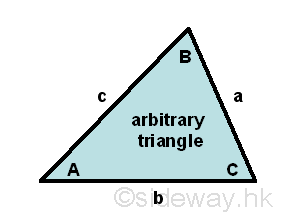The law of sines or sine rule are related to the sine functions of angles included in a triangle and the sides of the triangle. The Law of sines states that for a given arbitrary triangle with angle-side opposite pairs (A,a), (B,b) and (C,c), the ratios of the side opposite to an angle to the corresponding sine function of the angle are equal to a constant. Imply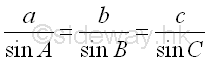There are three cases to be considered in proving the law of sines for the three different types of angled triangles. The acute angled triangle with all the internal angles are less than π/2. The right angled triangle with one of the internal angles is equal to π/2. The obtuse angled triangle with one of the internal angles is greater than π/2 but less than π.

For the first case, an acute triangle ΔABC with angle-side opposite pairs (A,a), (B,b) and (C,c) and all the internal angles less than π/2, three altitudes from vertexes A, B, and C can be constructed to the side opposite of the acute triangle.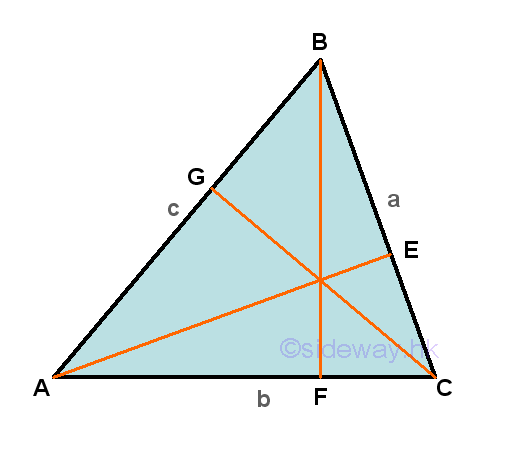Each altitude can divide the triangle into two paired right-angled triangles with a common side accordingly in a similar way. For example, ΔABC is divided by altitude BF to form two triangles  ΔBAF and ΔBCF. Let the altitude BF be the side opposite of the sine functions of the two corresponding angles ∠BAF and ∠BCF, then according to the definition of sine function, the side opposite of angle ∠BCF will be the hypotenuse of the right angled triangle ΔBAF and the side opposite of angle ∠BAF will be the hypotenuse of the right angled triangle ΔBCF respectively. That is sin A=BF/c and sin C=BF/a, imply BF=c(sin A)=a(sin C), and therefore a/sin A=c/sin C. Repeating the steps by considering another altitude, for example AE. Then ΔABC is divided by altitude AE to form two right-angled triangles  ΔACEand ΔABE. Let the altitude AE be the side opposite of the sine functions of the two corresponding angles ∠ACE and ∠ABE, then according to the definition of sine function, the side opposite of angle ∠ACE will be the hypotenuse of the right angled triangle ΔABE and the side opposite of angle ∠ABE will be the hypotenuse of the right angled triangle ΔACE respectively. That is sin C=AE/b and sin B=AE/c, imply AE=b(sin C)=c(sin B), and therefore b/sin B=c/sin C. Equating two equations then a/sin A=b/sin B=c/sin C. Imply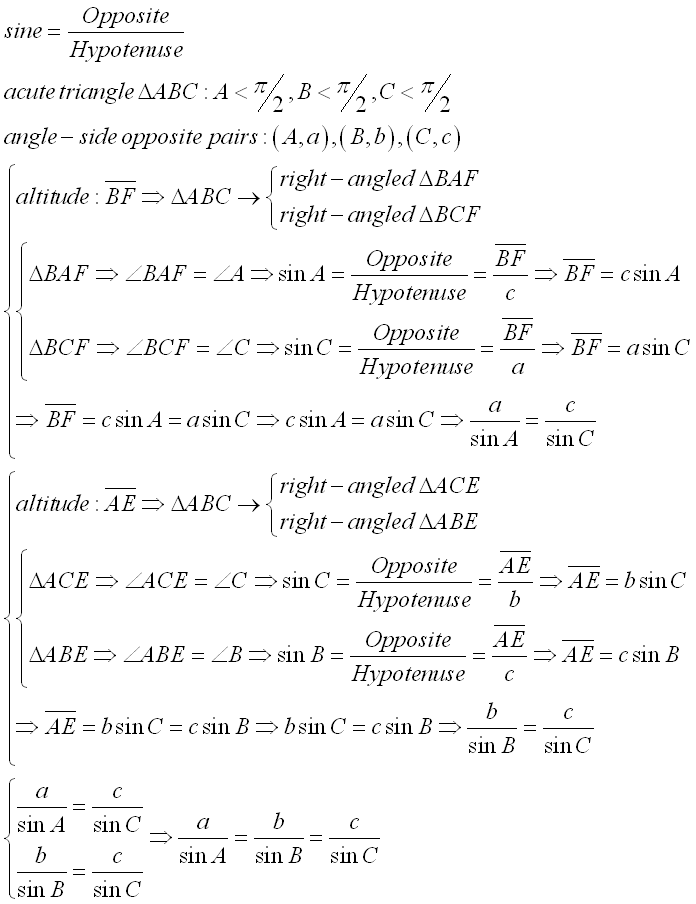For the second case, an obtuse triangle ΔABC with angle-side opposite pairs (A,a), (B,b) and (C,c) and one of the internal angles, A is greater than π/2 but less than π. Only one of the three altitudes which is drawn from vertex A can be constructed to the side opposite of the obtuse triangle, while the other two altitudes lie outside the obtuse triangle.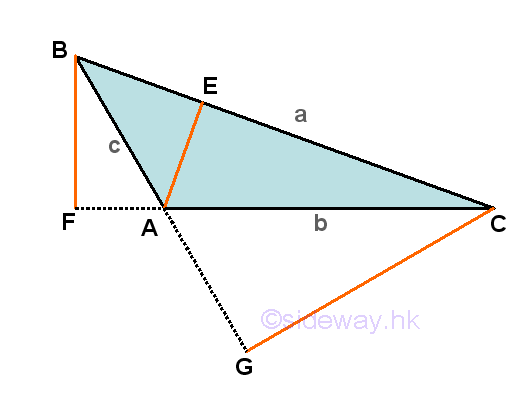Similarly, ΔABC is divided by altitude AE to form two triangles  ΔABE and ΔACE. Let the altitude AE be the side opposite of the sine functions of the two corresponding angles ∠ABE and ∠ACE, then according to the definition of sine function, the side opposite of angle ∠ACE will be the hypotenuse of the right angled triangle ΔABE and the side opposite of angle ∠ABE will be the hypotenuse of the right angled triangle ΔACE respectively. That is sin B=AE/c and sin C=AE/b, imply AE=c(sin B)=b(sin C), and therefore b/sin B=c/sin C. Repeating the steps by considering another altitude, for example BF. Although ΔABC is not divided by altitude BF, the dropping of the altitude from vertix B also forms two new right-angled triangles,   ΔBAF and ΔBCF. Let the altitude BF be the side opposite of the sine functions of the two corresponding angles ∠BAF and ∠BCF, then according to the definition of sine function, the side opposite of angle ∠BCA will be the hypotenuse of the right angled triangle ΔBAF and the side opposite of angle ∠BAC will be the hypotenuse of the right angled triangle ΔBCF respectively. That is sin(∠BAF)=BF/c and sin C=BF/a, imply BF=c(sin(∠BAF))=a(sin C). Since ∠BAF=π-∠BAC=π-A, then sin(∠BAF)=sin(π-A), by reduction formula, sin(∠BAF)=sin A. And therefore c(sin A)=a(sin C), imply c/sin C=a/sin A. Equating two equations then a/sin A=b/sin B=c/sin C. Imply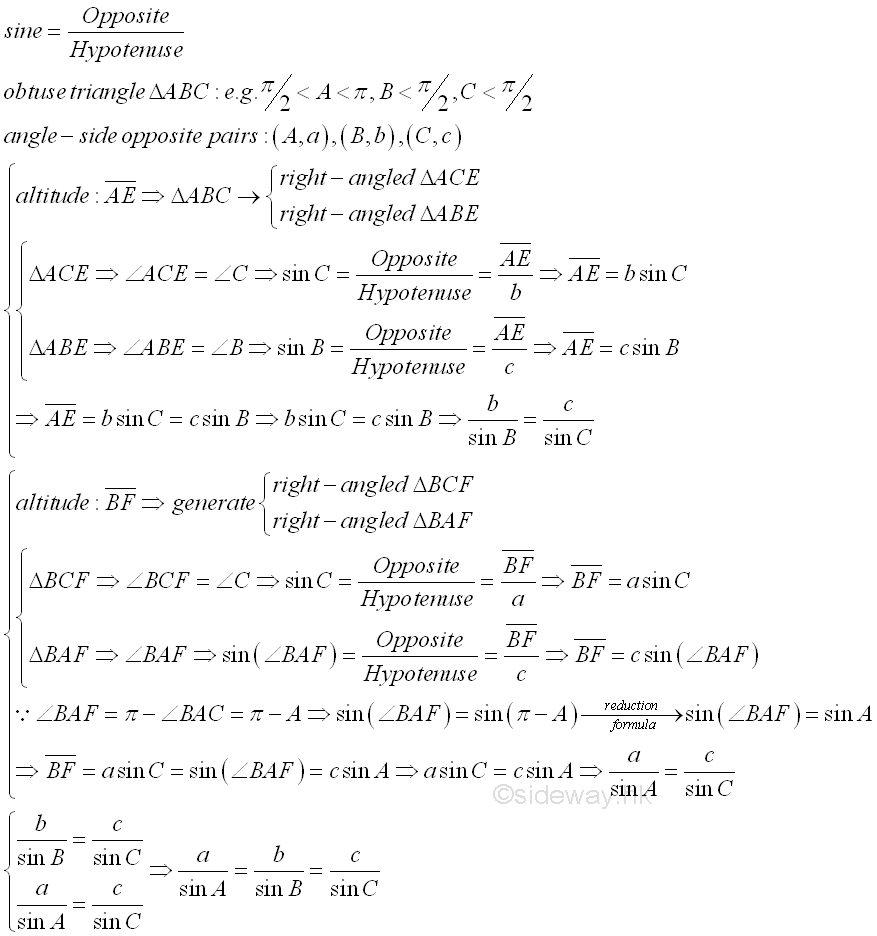For the third case, an right-angled triangle ΔABC with angle-side opposite pairs (A,a), (B,b) and (C,c) and one of the internal angles, A is equal to π/2. Only one of the three altitudes which is drawn from vertex A can be constructed to the side opposite of the right-angled triangle, while the other two altitudes lie on the sides of the right-angle triangle.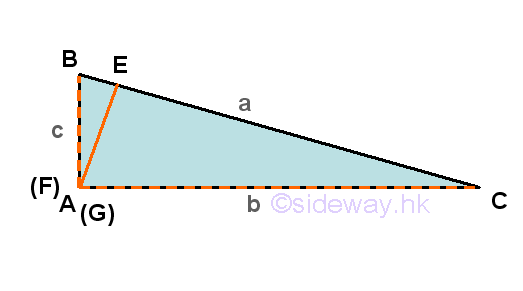Similarly, ΔABC is divided by altitude AE to form two triangles  ΔABE and ΔACE. Let the altitude AE be the side opposite of the sine functions of the two corresponding angles ∠ABE and ∠ACE, then according to the definition of sine function, the side opposite of angle ∠ACE will be the hypotenuse of the right angled triangle ΔABE and the side opposite of angle ∠ABE will be the hypotenuse of the right angled triangle ΔACE respectively. That is sin B=AE/c and sin C=AE/b, imply AE=c(sin B)=b(sin C), and therefore b/sin B=c/sin C. Repeating the steps by considering another altitude, for example BF. Although ΔABC is not divided by altitude BF, the dropping of the altitude from vertix B is exactly the side of the right-angled triangle,   ΔABC. According to the definition of sine function, the sine function of angle ∠BCA or sin C is sin C=c/a, imply a=c/sin C and the sine function of angle ∠ABC or sin B is sin B=b/a, imply a=b/sin B, therefore a=c/sin C=b/sin B. Since sin A = sin π/2 is equal 1, therefore a/sin A=b/sin B=c/sin C. Imply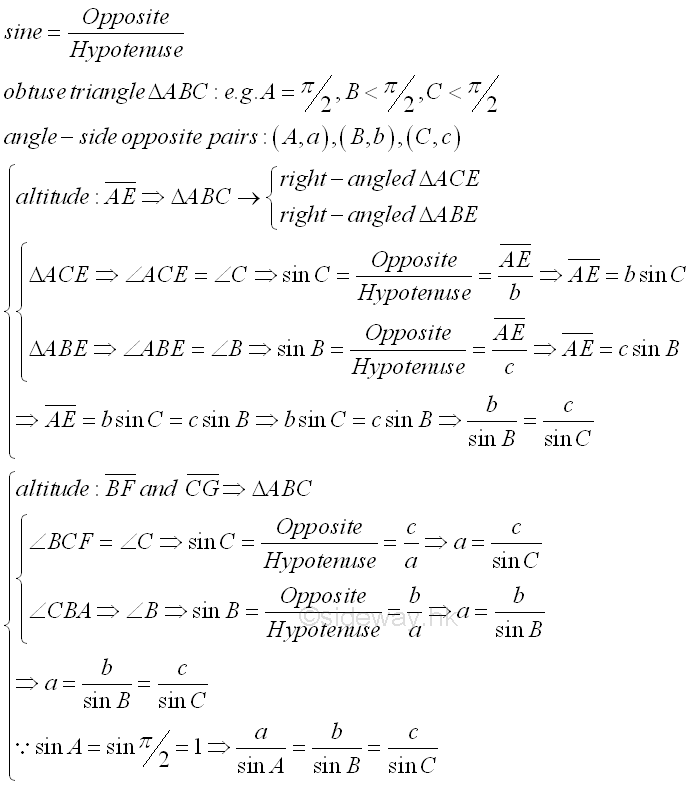Since the internal angle sum of a triangle is equal to π, the law of sines can therefore always be used to determine the remaining sides of a triangle when two angles and a side are known because the third angle can be uniquely determined using the equation C= π-A-B and the shape of the triangle is also completely determined. So, the shape of a triangle with the known forms of Angle-Angle-Side (AAS) and Angle-Side-Angle (ASA) can be determined by the law of sines. But for the case of given one angle and  two sides, the application of the law will have problem. If there is no ratio relationship in the three given dimensions, i.e. Side-Angle-Side (SAS), the law of sines cannot be applied directly. If the three given measures are Side-Side-Angle (SSA) or Angle-Side-Side (ASS), there always exists a paired angle-side opposite ratio, and the angle of the corresponding side opposite can then be found. However, as from the reduction formula, the sine function of an angle is equal to the sine function of π-the same angle if the given angle is less than π/2. In other words, there may have two distinct triangles which satisfy the given criteria. Unless when the given angle is greater or equal to π/2, as the internal angle sum of a triangle is equal to π, the other two unknown angles can only be angles smaller than π/2 and the shape of the triangle is fixed.  In fact, there are four possibilities in the Side-Side-Angle (SSA) cases when the given angle is less than π/2.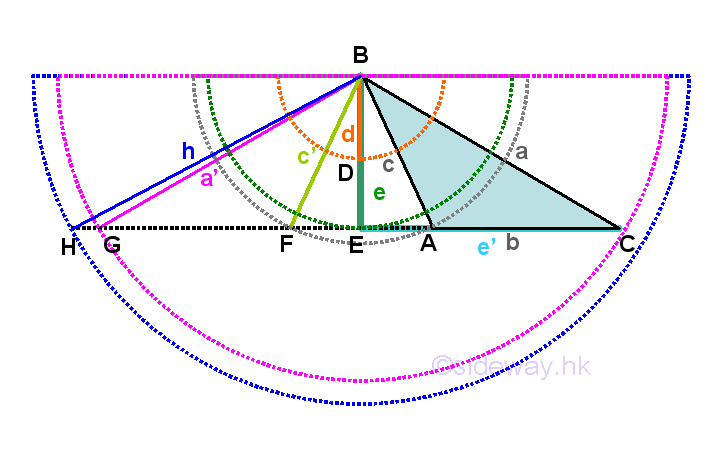If the given criteria is either abA or acA with an obtuse angle A, the shape of the triangle must be an obtuse triangle ΔABC. If the given criteria is either aeE or ae'E with a right angle E, then the shape of the triangle must be a right-angled triangle ΔECB. If the given criteria is either caC or cbC with angle C less the π/2, then the side c may fall into four cases. For the side-side-angle caC condition, let BE be e and is equal to a(sin C).  For the first case, the given side c is smaller than a(sin C). If there exists a triangle, from the law of sines, c/sin C=a/sin A, imply sin A=a(sin C)/c, since c<a(sin C), imply sin A>1. Sine function greater than one is impossible and no triangle can be constructed because the side c<e is too short to form a triangle.  For the second case, the given side c is equal to a(sin C). If there exists a triangle, from the law of sines, c/sin C=a/sin A, imply sin A=a(sin C)/c, since c=a(sin C), imply sin A=1. Sine function eqaul to one is possible when A is equal to π/2, a right-angled triangle can be constructed as required. For the third case, the given side a>c>a(sin C). If there exists a triangle, from the law of sines, c/sin C=a/sin A, imply sin A=a(sin C)/c, since a>c>a(sin C), imply sin C<sin A<1. Sine function A greater than sin C and less than one is possible, from reduction formula, there are two solution for angle A, that is the acute angle A and the supplement of angle A, i.e. π-A. When angle A is an acute angle, since angle C is an acute angle, angles A plus C is always less than π. When angle A is the supplement of angle A, since sin C<sin A, angle A is great than angle C, therefore sum of angles π-A+C is always less than  π also. With the sum of angles A and C is not equal to π, a triangle can be constructed and therefore two distinct triangles exist. For the fourth case, the given side c is greater than or equal to a. If there exists a triangle, from the law of sines, c/sin C=a/sin A, imply sin A=a(sin C)/c, since a≤c, imply sin A≤sin C. Since angle C is an acute angle, angle A must be an acute angle also. And therefore only one acute triangle can be constructed. Imply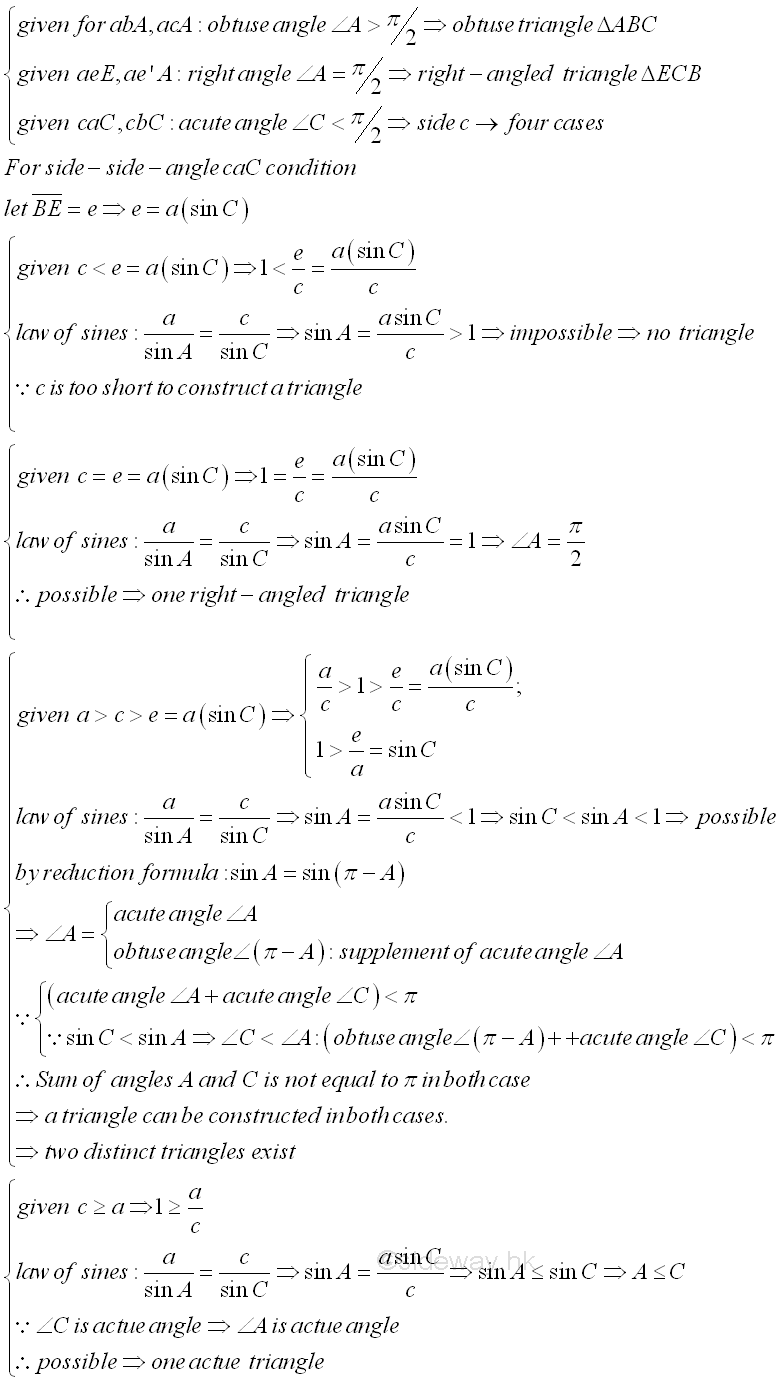ID: 130600011 Last Updated: 13/6/2013 Revision: 1 Ref:References

1. B. Joseph, 1978
2. Ayres, F. JR, Moyer, R.E., 1999
3. Hopkings, W., 1833Home 5

Management

HBR 3

Information

Recreation

Culture

Chinese 1097

English 337

Computer

Hardware 149

Software

Application 187

Numeric 19

Programming

Web 757

CSS 1

HTML

Knowledge Base

OS 389

MS Windows

Knowledge

Mathematics

Algebra 20

Trigonometry 18

Geometry 18

Calculus 67

Engineering

Mechanical

Rigid Bodies

Statics 92

Dynamics 37

Control

Physics

Electric 10